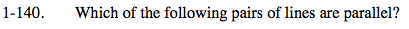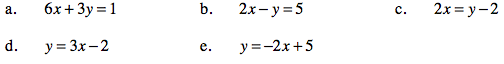### Home > PC > Chapter 1 > Lesson 1.4.1 > Problem1-140

1-140.
1. Which of the following pairs of lines are parallel? Homework Help ✎

1. 6x + 3y = 1

2. 2xy = 5

3. 2x = y − 2

4. y = 3x − 2

5. y = −2x + 5Put each equation in the y-form and compare their slopes. Parallel slopes will be the same.